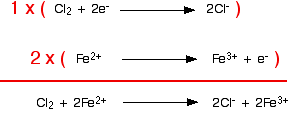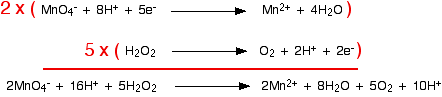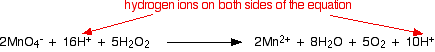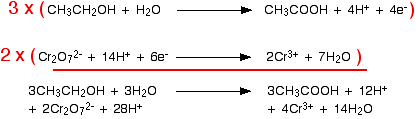Need help? Email help@shout.education

# Writing Ionic Equations for Redox Reactions

This page explains how to work out electron-half-reactions for oxidation and reduction processes, and then how to combine them to give the overall ionic equation for a redox reaction. This is an important skill in inorganic chemistry.

Don't worry if it seems to take you a long time in the early stages. It is a fairly slow process even with experience. Take your time and practise as much as you can.

## Electron Half-equations

### What is an Electron Half-equation?

When magnesium reduces hot copper(II) oxide to copper, the ionic equation for the reaction is:

\text{Cu}^{2+} + \text{Mg} \longrightarrow \text{Cu} + \text{Mg}^{2+}

Note: I am going to leave out state symbols in all the equations on this page. This topic is awkward enough anyway without having to worry about state symbols as well as everything else. Practice getting the equations right, and then add the state symbols in afterwards if your examiners are likely to want them.

How do you know whether your examiners will want you to include them? The best way is to look at their mark schemes. You should be able to get these from your examiners' website. There are links on the syllabuses page for students studying for UK-based exams.

You can split the ionic equation into two parts, and look at it from the point of view of the magnesium and of the copper(II) ions separately. This shows clearly that the magnesium has lost two electrons, and the copper(II) ions have gained them.

\begin{aligned} \text{Mg} &\longrightarrow \text{Mg}^{2+} + \color{#467abf}{2\text{e}^-} \\ \\ \text{Cu}^{2+} + \color{#467abf}{2\text{e}^-} &\longrightarrow \text{Cu} \end{aligned}

These two equations are described as "electron half-equations" or "half-equations" or "ionic half-equations" or "half-reactions" – lots of variations all meaning exactly the same thing!

Any redox reaction is made up of two half-reactions: in one of them electrons are being lost (an oxidation process) and in the other one those electrons are being gained (a reduction process).

Note: If you aren't happy about redox reactions in terms of electron transfer, you MUST read the introductory page on redox reactions before you go on.

### Working Out Electron Half-equations and Using Them to Build Ionic Equations

In the example above, we've got at the electron half-equations by starting from the ionic equation and extracting the individual half-reactions from it. That's doing everything entirely the wrong way round!

In reality, you almost always start from the electron half-equations and use them to build the ionic equation.

#### Example 1: The reaction between chlorine and iron(II) ions

Chlorine gas oxidises iron(II) ions to iron(III) ions. In the process, the chlorine is reduced to chloride ions.

You would have to know this, or be told it by an examiner. In building equations, there is quite a lot that you can work out as you go along, but you have to have somewhere to start from!

You start by writing down what you know for each of the half-reactions. In the chlorine case, you know that chlorine (as molecules) turns into chloride ions:

\text{Cl}_2 \longrightarrow \color{#467abf}{\text{Cl}^-}

The first thing to do is to balance the atoms that you have got as far as you possibly can:

\text{Cl}_2 \longrightarrow \color{#467abf}{2}\text{Cl}^-

ALWAYS check that you have the existing atoms balanced before you do anything else. If you forget to do this, everything else that you do afterwards is a complete waste of time!

Now you have to add things to the half-equation in order to make it balance completely.

All you are allowed to add are:

• electrons
• water
• hydrogen ions (unless the reaction is being done under alkaline conditions – in which case, you can add hydroxide ions instead)

In the chlorine case, all that is wrong with the existing equation that we've produced so far is that the charges don't balance. The left-hand side of the equation has no charge, but the right-hand side carries 2 negative charges.

That's easily put right by adding two electrons to the left-hand side. The final version of the half-reaction is:

\text{Cl}_2 + \color{#467abf}{2\text{e}^-} \longrightarrow 2\text{Cl}^-

Now you repeat this for the iron(II) ions. You know (or are told) that they are oxidised to iron(III) ions. Write this down:

\text{Fe}^{2+} \longrightarrow \color{#467abf}{\text{Fe}^{3+}}

The atoms balance, but the charges don't. There are 3 positive charges on the right-hand side, but only 2 on the left.

You need to reduce the number of positive charges on the right-hand side. That's easily done by adding an electron to that side:

\text{Fe}^{2+} \longrightarrow \text{Fe}^{3+} + \color{#467abf}{\text{e}^-}

Combining the half-reactions to make the ionic equation for the reaction

What we've got at the moment is this:

\begin{aligned} \text{Cl}_2 + \overbrace{\color{#467abf}{2\text{e}^-}}^{\clap{\color{#467abf}{\text{this reaction needs 2 electrons...}}{}}} &\longrightarrow 2\text{Cl}^- \\ \\ \text{Fe}^{2+} &\longrightarrow \text{Fe}^{3+} + \underbrace{\color{#00CC99}{\text{e}^-}}_{\clap{\color{#00CC99}{\text{...but this one only produces 1 electron}}}{}} \end{aligned}

It is obvious that the iron reaction will have to happen twice for every chlorine molecule that reacts. Allow for that, and then add the two half-equations together.But don't stop there!! Check that everything balances – atoms and charges. It is very easy to make small mistakes, especially if you are trying to multiply and add up more complicated equations.

You will notice that I haven't bothered to include the electrons in the added-up version. If you think about it, there are bound to be the same number on each side of the final equation, and so they will cancel out. If you aren't happy with this, write them down and then cross them out afterwards!

#### Example 2: The reaction between hydrogen peroxide and manganate(VII) ions

The first example was a simple bit of chemistry which you may well have come across. The technique works just as well for more complicated (and perhaps unfamiliar) chemistry.

Manganate(VII) ions, MnO4-, oxidise hydrogen peroxide, H2O2, to oxygen gas. The reaction is done with potassium manganate(VII) solution and hydrogen peroxide solution acidified with dilute sulfuric acid.

During the reaction, the manganate(VII) ions are reduced to manganese(II) ions.

\text{H}_2\text{O}_2 \longrightarrow \color{#467abf}{\text{O}_2}

All you are allowed to add to this equation are water, hydrogen ions and electrons. If you add water to supply the extra hydrogen atoms needed on the right-hand side, you will mess up the oxygens again – that's obviously wrong!

Add two hydrogen ions to the right-hand side.

\text{H}_2\text{O}_2 \longrightarrow \text{O}_2 + \color{#467abf}{2\text{H}^+}

Now all you need to do is balance the charges. You would have to add 2 electrons to the right-hand side to make the overall charge on both sides zero.

\text{H}_2\text{O}_2 \longrightarrow \text{O}_2 + 2\text{H}^+ + \color{#467abf}{2\text{e}^-}
##### Now for the manganate(VII) half-equation:

You know (or are told) that the manganate(VII) ions turn into manganese(II) ions. Write that down.

{\text{MnO}_4}^- \longrightarrow \color{#467abf}{\text{Mn}^{2+}}

The manganese balances, but you need four oxygens on the right-hand side. These can only come from water – that's the only oxygen-containing thing you are allowed to write into one of these equations in acid conditions.

{\text{MnO}_4}^- \longrightarrow \text{Mn}^{2+} + \color{#467abf}{4\text{H}_2\text{O}}

By doing this, we've introduced some hydrogens. To balance these, you will need 8 hydrogen ions on the left-hand side.

{\text{MnO}_4}^- + \color{#467abf}{8\text{H}^+} \longrightarrow \text{Mn}^{2+} + 4\text{H}_2\text{O}

Now that all the atoms are balanced, all you need to do is balance the charges. At the moment there are a net 7+ charges on the left-hand side (1- and 8+), but only 2+ on the right. Add 5 electrons to the left-hand side to reduce the 7+ to 2+.

{\text{MnO}_4}^- + 8\text{H}^+ + \color{#467abf}{5\text{e}^-} \longrightarrow \text{Mn}^{2+} + 4\text{H}_2\text{O}

This is the typical sort of half-equation which you will have to be able to work out.

• Balance the atoms apart from oxygen and hydrogen.
• Balance the oxygens by adding water molecules.
• Balance the hydrogens by adding hydrogen ions.
• Balance the charges by adding electrons.

Combining the half-reactions to make the ionic equation for the reaction

The two half-equations we've produced are:

\begin{aligned} {\text{MnO}_4}^- + 8\text{H}^+ + \overbrace{\color{#467abf}{5\text{e}^-}}^{\clap{\color{#467abf}{\text{this reaction needs 5 electrons...}}{}}} &\longrightarrow \text{Mn}^{2+} + 4\text{H}_2\text{O} \\ \\ \text{H}_2\text{O}_2 &\longrightarrow \text{O}_2 + 2\text{H}^+ + \underbrace{\color{#00CC99}{2\text{e}^-}}_{\clap{\color{#00CC99}{\text{...but this one only produces 2 electrons}}}{}} \end{aligned}

You have to multiply the equations so that the same number of electrons are involved in both.In this case, everything would work out well if you transferred 10 electrons.But this time, you haven't quite finished. During the checking of the balancing, you should notice that there are hydrogen ions on both sides of the equation:You can simplify this down by subtracting 10 hydrogen ions from both sides to leave the final version of the ionic equation – but don't forget to check the balancing of the atoms and charges!

2{\text{MnO}_4}^- + 6\text{H}^+ + 5\text{H}_2\text{O}_2 \longrightarrow 2\text{Mn}^{2+} + 8\text{H}_2\text{O} + 5\text{O}_2

You will often find that hydrogen ions or water molecules appear on both sides of the ionic equation in complicated cases built up in this way. Always check, and then simplify where possible.

#### Example 3: The oxidation of ethanol by acidified potassium dichromate(VI)

This technique can be used just as well in examples involving organic chemicals. Potassium dichromate(VI) solution acidified with dilute sulfuric acid is used to oxidise ethanol, CH3CH2OH, to ethanoic acid, CH3COOH.

The oxidising agent is the dichromate(VI) ion, Cr2O72-. This is reduced to chromium(III) ions, Cr3+.

We'll do the ethanol to ethanoic acid half-equation first. Using the same stages as before, start by writing down what you know:

\text{CH}_3\text{CH}_2\text{OH} \longrightarrow \text{CH}_3\text{COOH}

Balance the oxygens by adding a water molecule to the left-hand side:

\text{CH}_3\text{CH}_2\text{OH} + \color{#467abf}{\text{H}_2\text{O}} \longrightarrow \text{CH}_3\text{COOH}

Add hydrogen ions to the right-hand side to balance the hydrogens:

\text{CH}_3\text{CH}_2\text{OH} + \text{H}_2\text{O} \longrightarrow \text{CH}_3\text{COOH} + \color{#467abf}{4\text{H}^+}

And finally balance the charges by adding 4 electrons to the right-hand side to give an overall zero charge on each side:

\text{CH}_3\text{CH}_2\text{OH} + \text{H}_2\text{O} \longrightarrow \text{CH}_3\text{COOH} + 4\text{H}^+ + \color{#467abf}{4\text{e}^-}

The dichromate(VI) half-equation contains a trap which lots of people fall into!

Start by writing down what you know:

{\text{Cr}_2\text{O}_7}^{2-} \longrightarrow \color{#467abf}{\text{Cr}^{3+}}

What people often forget to do at this stage is to balance the chromiums. If you don't do that, you are doomed to getting the wrong answer at the end of the process! When you come to balance the charges you will have to write in the wrong number of electrons – which means that your multiplying factors will be wrong when you come to add the half-equations. A complete waste of time!

{\text{Cr}_2\text{O}_7}^{2-} \longrightarrow \color{#467abf}{2}\text{Cr}^{3+}
{\text{Cr}_2\text{O}_7}^{2-} \longrightarrow 2\text{Cr}^{3+} + \color{#467abf}{7\text{H}_2\text{O}}
{\text{Cr}_2\text{O}_7}^{2-} + \color{#467abf}{14\text{H}^+} \longrightarrow 2\text{Cr}^{3+} + 7\text{H}_2\text{O}

Now all that needs balancing is the charges. Add 6 electrons to the left-hand side to give a net 6+ on each side.

{\text{Cr}_2\text{O}_7}^{2-} + 14\text{H}^+ + \color{#467abf}{6\text{e}^-} \longrightarrow 2\text{Cr}^{3+} + 7\text{H}_2\text{O}

Combining the half-reactions to make the ionic equation for the reaction

What we have so far is:

\begin{aligned} \text{CH}_3\text{CH}_2\text{OH} + \text{H}_2\text{O} &\longrightarrow \text{CH}_3\text{COOH} + 4\text{H}^+ + 4\text{e}^- \\ \\ {\text{Cr}_2\text{O}_7}^{2-} + 14\text{H}^+ + 6\text{e}^- &\longrightarrow 2\text{Cr}^{3+} + 7\text{H}_2\text{O} \end{aligned}

What are the multiplying factors for the equations this time? The simplest way of working this out is to find the smallest number of electrons which both 4 and 6 will divide into – in this case, 12. That means that you can multiply one equation by 3 and the other by 2.

Note: Don't worry too much if you get this wrong and choose to transfer 24 electrons instead. All that will happen is that your final equation will end up with everything multiplied by 2. Your examiners might well allow that.

The multiplication and addition looks like this:Now you will find that there are water molecules and hydrogen ions occurring on both sides of the ionic equation. You can simplify this to give the final equation:

3\text{CH}_3\text{CH}_2\text{OH} + 2{\text{Cr}_2\text{O}_7}^{2-} + 16\text{H}^+ \longrightarrow 3\text{CH}_3\text{COOH} + 4\text{Cr}^{3+} + 11\text{H}_2\text{O}

Note: You have now seen a cross-section of the sort of equations which you could be asked to work out. Now you need to practice so that you can do this reasonably quickly and very accurately! Aim to get an averagely complicated example done in about 3 minutes.

If you want a few more examples, and the opportunity to practice with answers available, you might be interested in looking in chapter 1 of my book on Chemistry Calculations.

### Reactions Done Under Alkaline Conditions

Working out half-equations for reactions in alkaline solution is decidedly more tricky than those above. You are less likely to be asked to do this at this level (UK A-level and its equivalents), and for that reason I've covered these on a separate page (link below). It would be worthwhile checking your syllabus and past papers before you start worrying about these!